## Diameter of oleic acid molecule

### Experiment number : 2083

• #### Goal of experiment

• To show that one can easily measure the diameter of something as small as a molecule, which is approximately 10−9 to 10−10 m.
• To measure the diameter of a molecule of the oleic acid in particular.
• #### Theory

Spread some cork powder onto the water surface and drop a droplet of oleic acid and kerosene solution onto it. Kerosene will quickly evaporate and the oleic acid will spread on the water surface. It will create a thin circular layer that is only one molecule thick (so called monomolecular).

This layer can be called a cylinder whose height is the diameter of one molecule dm. The radius of the base of the cylinder will be labeled r.

The volume of the cylinder can be calculated from

$V = πr^{2}·d_{\mathrm{m}}.\tag{1}$

This cylinder is made purely by oleic acid, thus its volume is equal to the volume of the oleic acid in the droplet of the solution.

The volume of the droplet can be calculated like this:

$V_{droplet} = \frac{1}{x} (\mathrm{ml}) = \frac{10^{−3}}{x} (\mathrm{l}),$

where x is the amount of droplets in 1 ml of the solution.

The oleic acid makes up only a part of the droplet’s volume. In our case, we use a solution with the oleic acid – kerosene ratio of 1:2000. The volume of the oleic acid in one droplet can be determined as

$V = \frac{1}{2000}V_{droplet} = \frac{1}{2000}\frac{10^{−3}}{x} (\mathrm{l}) = \frac{10^{−6}}{2x} (\mathrm{l}).$

Since we want to calculate the diameter of the molecule in meters, we want to calculate the volume in cubic meters (m3)

$V = \frac{10^{−9}}{2x} (\mathrm{m}^3).\tag{2}$

We can formulate a relation for the molecule’s diameter dm from relations (1) and (2)

$d_{\mathrm{m}} = \frac{10^{−9}}{2πr^{2}·x}.\tag{3}$
• #### Equipment

• pipette or medical dropper

• volumetric tube

• oleic acid

• kerosene or medical gasoline

• cork sponge

• bowl with diameter of at least 35 cm

• water

• meter or longer ruler

Preparation

Prepare a solution of oleic acid and kerosene in 1:2000 ratio.

Create powder from cork using a file.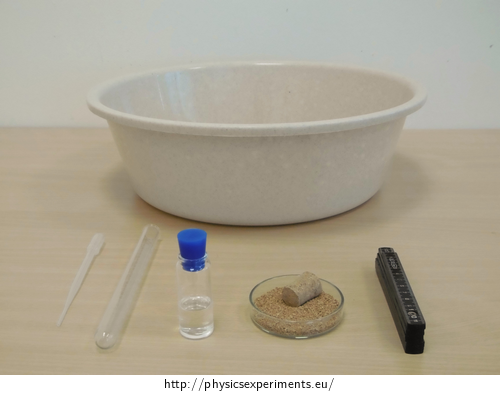• #### Procedure

1. Find out how many droplets are there in 1 ml using a pipette and volumetric tube (labeled as x in theoretical part).

2. Pour water into the bowl, wait till the surface calms down and then cover it with cork powder.

3. Drop one droplet of prepared solution of oleic acid and kerosene into the middle of the bowl.

4. Kerosene will quickly evaporate and the oleic acid will create a circular layer on the surface that will be bounded by cork powder.

5. Measure the diameter of the circle at several places using the meter. Find the arithmetic average of these values.

6. Calculate the diameter of the molecule of oleic acid using the relation from the theoretical part.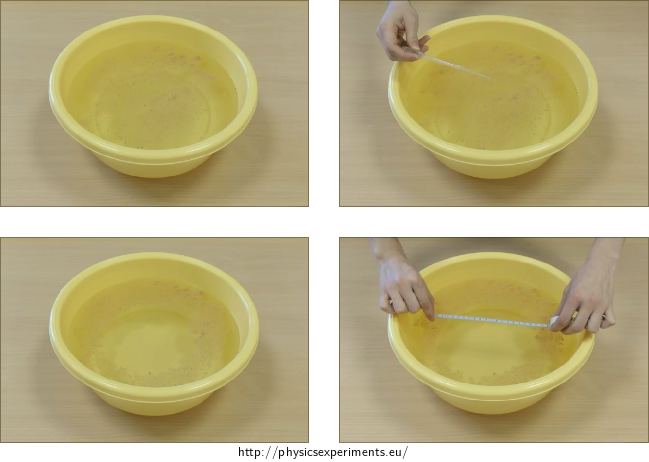• #### Example of results

We found out that the volume of 1 ml is 24 droplets. The circular layer on the surface has diameter of d = 0.19 m. Accordingly to relation (3), we calculated that the diameter of the molecules of oleic acid is dm = 8.1·10−10 m.

Though this way of measuring isn’t very precise, the result isn’t too far from value we calculated using a more precise way in task Diameter of Oleic Acid Molecule – there, we calculated the diameter to be d = 9.07·10−10 m.

• #### Technical notes

• It is necessary that the cork powder is fine enough. Using a grater would result into too big parts of cork and those are too heavy for the experiment to work.

• #### Methodical notes

• The preparation of the solution should be done in advance.

• This experiment can be done as a laboratory work. You can do a precise calculation of the molecule’s diameter beforehand using molar weight and density (see task Diameter of Oleic Acid Molecule).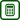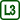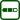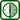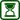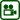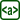×Original source: Svoboda, E. a kol.: Pokusy z fyziky na střední škole 2. Prometheus, Praha 1997